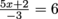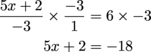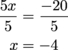Study Guide

# Basic Algebra - Solving More Complex Equations

## Solving More Complex Equations

This multi-step business may be a bit more complicated than what we've already been doing, but it's nothing we can't handle. It just involves three or more of the same kinds of steps.

We've already seen how to solve for x in an equation that has two operations, like addition and multiplication. Doing PEMDAS backwards, we usually get rid of the numbers being added and subtracted first, and then the numbers being multiplied and divided. The key word here is usually because this ain't no hard-and-fast math rule.

This section will show a few equations where that rule won't work. For problems like these (and any math equation, really), we're going to get rid of the numbers furthest from the x first, and then move in on the x, eliminating numbers as we go. To solve for x in these equations, we need to start with the last operation that we'd do if we were to plug in a value for x.

In the equation, we have three numbers connected to x on the left side of the equal sign: x is being multiplied by 5, added to 2, and then divided by -3. To undo all of that mumbo-jumbo, we need to work it backwards.

The last operation on the list is division by -3, and the number furthest from the x is -3. So we're going to get rid of that "divide by -3" first by multiplying both sides by -3. (Notice we convert the integer -3 into a fraction to be able to multiply it on the left side of the equation.)Next, we need to get rid of the "add 2" by subtracting 2 from both sides.

5x + 2 – 2 = -18 – 2
5x = -20

Finally, we divide by 5.There's our answer.

Sometimes equations have more than one term with a variable. In that case, we need to combine all the like terms and then solve.

For example, in the equation 3x – 2 + 6x = 16, there are two terms with an x: 3x and 6x. We can combine these to get a simpler equation.

3x – 2 + 6x = 16
9x – 2 = 16

Now we can solve for x by adding 2 and dividing by 9.

9x – 2 = 16
9x – 2 + 2 = 16 + 2
9x = 18
x = 2

Shmoopalicious: the answer is x = 2.